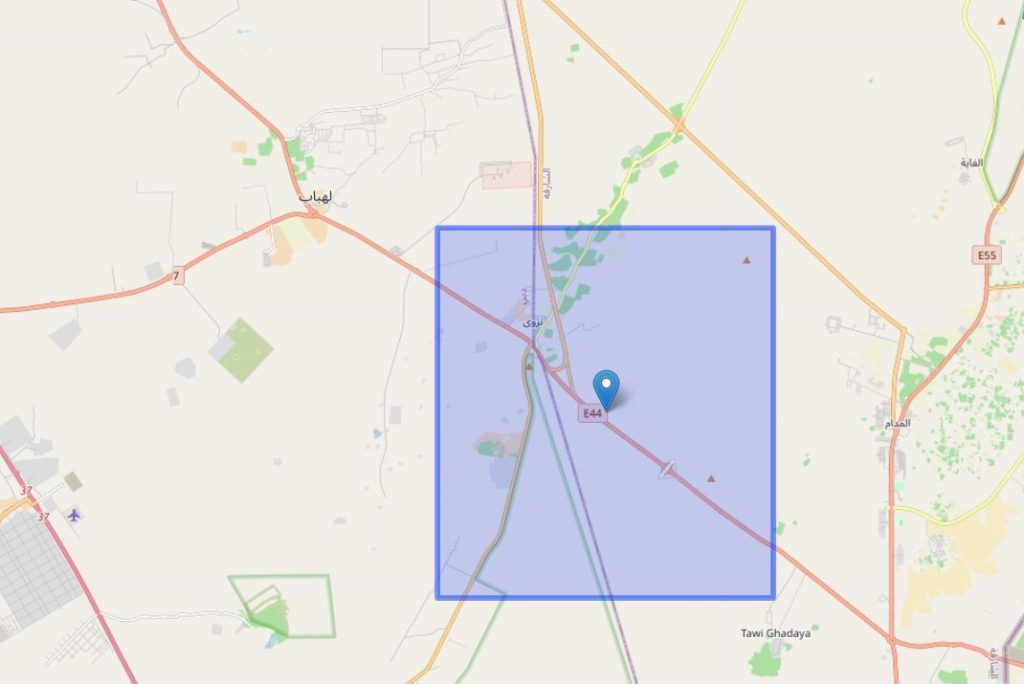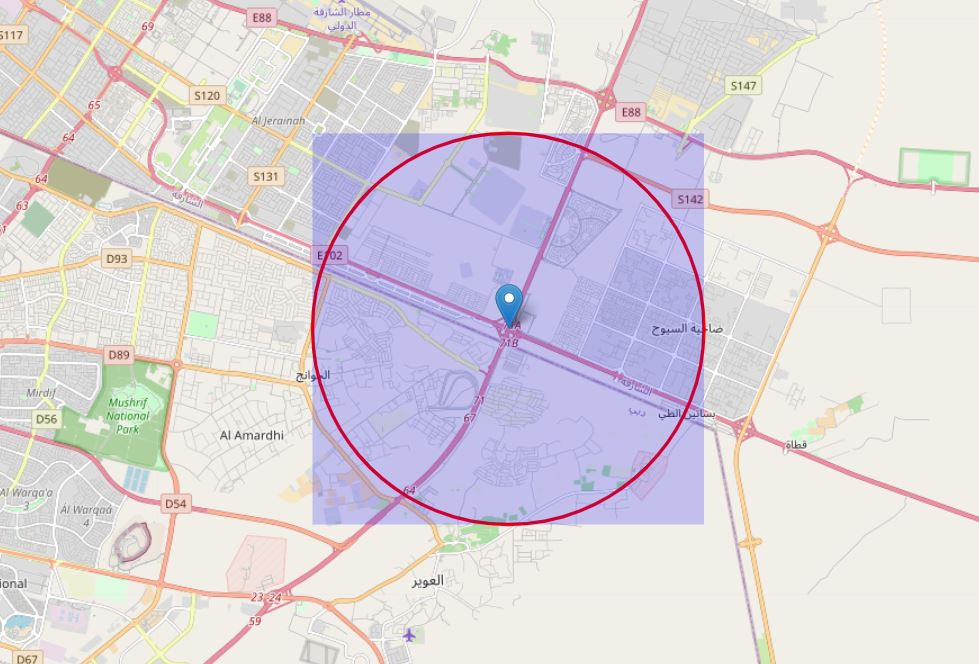# Javascript to Create Rectangle based on a Longitude / Latitude in leaflet

By | September 13, 2020

Scenario : you need to create a rectangle in leaflet based on a point for which you know the long / lat.```function DrawRectangle(lat, lng) {

var km = 12;

// number of km per degree = ~111km (111.32 in google maps, but range varies  between 110.567km at the equator and 111.699km at the poles)
// 1km in degree = 1 / 111.32km = 0.0089
// 1m in degree = 0.0089 / 1000 = 0.0000089
var coef = km * 0.0089 / 2;

var latref = lat;
var lonref = lng;

var mx_lat = latref + coef;
var mxm_lat = latref - coef;
var mx_lon = lonref + coef;
var mxm_lon = lonref - coef;

var southWest = L.latLng(mxm_lat, mxm_lon),
northEast = L.latLng(mx_lat, mx_lon),
bounds = L.latLngBounds(southWest, northEast);

}
```

I realized that above is not exactly a square and below is the one which draws a square from circle.

```function DrawCircle(lat, lng) {

var circle = L.circle([lat, lng], {
color: "red",
fillColor: "#f03",
fillOpacity: 0.0,